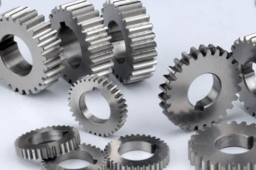# Tutorials - How to make a Involute Spur GearTutorials - How to make a Involute Spur Gear

Initially Take a Note on spur gear dimensions as follows

Module = 4mm
Number of teeth =39
Pitch Circle Diameter =156 mm
Pressure Angle =20 Deg
Root Diameter = 149.70 mm
Dedendum Circle Diameter =130 mm
Hole size 80 mm
Tooth thickness =6.28mm
Base Circle Diameter =156cos(20)=146.592mm

Step 1

• Select the Front plane

• Draw 130mm (Dedendum Circle Diameter) Diameter circle and a Hole 80mm Diameter circle

• Extrude 50 mm

Step 2

Draw three circles vice the diameters are
• Addendum Circle Diameter =167.4 mm,
• Pitch Circle Diameter =156 mm,
• Base Circle Diameter =156cos(20)=146.592mm

Step 3 For involute curve we need to put some parametric equations as follows
Follow the procedure and paste the equations

Menu bar - Insert - Model datum – Curve - In the menu manager - From equation - Done
Now the select the PRT_CSYS_DEF and Cartesian (a notepad box will open)
Just paste the following equations without modify anything..,

Angle=t*90
X=X_ins+(Cir_len*sin(Angle))
Y=Y_ins-(Cir_len*cos(Angle))
Z=0

(Note : Here 146.592/2 mm is Base Circle Radius)
File - Save - Close notepad -Preview - OK
Now you see the curve

Step 4
(Note : Tooth thickness=6.28mm on pitch circle)
• Again Go to sketcher using previous sketching plane (Take references before draw )
• Menu bar/right click Sketcher - References
• Pick the curve and the middle circle as references
Then draw a center point arc with radius of 6.28 mm on the middle circle and the starting point should start from the curve.

Take a point on the curve at middle
Exit Sketcher
Then
Datum point - Select the curve - offset = .50

Step 5

Now we make a datum plane passing through this point and the central axis. Then we reflect the curve about datum plane
Datum plane - Select the point and the axis - OK
Now Datum plane created.

Select the curve we draw via the formula and mirror it with reference to Datum plane

Now we use the curve to define the tooth profile

Steps
Sketcher - the create an entity from edge option from tool bar - Select the two curves

Sketch - References select the three circles. Select the references as shown in the figure.

Draw the two connecting lines and two center point arc as shown in figure.

Then trim the unnecessary lines to make the profile.

(Note : The profile must be closed at the end)
Finally extrude the profile and pattern it about the central axis.

Hide all the unnecessary curves

Well the Involute spur gear is formed successfully.